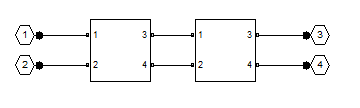## Description

Use the `cascade` object to represent cascaded networks of RF objects that are characterized by the components that make up the individual network. The following figure shows the configuration of a pair of cascaded networks.## Creation

### Syntax

``h = rfckt.cascade``
``h = rfckt.cascade('Ckts',value)``

### Description

````h = rfckt.cascade` returns a cascaded network object whose properties all have their default values.```

example

````h = rfckt.cascade('Ckts',value)` returns a cascaded network with elements specified in the name-value pair property `Ckts`.```

## Properties

expand all

Computed S-parameters, noise figure, OIP3, and group delay values. For more information, see Algorithms.

Data Types: `function_handle`

Circuit objects in network. All circuits must be 2-port. By default, this property is empty.

Data Types: `char`

Data Types: `char`

Number of ports of cascaded network. The default value is `2`.

Data Types: `double`

## Object Functions

 `analyze` Analyze RFCKT object in frequency domain `calculate` Calculate specified parameters for rfckt objects or rfdata objects `circle` Draw circles on Smith Chart `extract` Extract specified network parameters from rfckt object or data object `listformat` List valid formats for specified circuit object parameter `listparam` List valid parameters for specified circuit object `loglog` Plot specified circuit object parameters using log-log scale `plot` Plot circuit object parameters on X-Y plane `plotyy` Plot parameters of RF circuit or RF data on X-Y plane with two Y-axes `getop` Display operating conditions `polar` Plot specified object parameters on polar coordinates `semilogx` Plot RF circuit object parameters using log scale for x-axis `semilogy` Plot RF circuit object parameters using log scale for y-axis `smith` Plot circuit object parameters on Smith chart `write` Write RF data from circuit or data object to file `getz0` Calculate characteristic impedance of RFCKT transmission line object `read` Read RF data from file to new or existing circuit or data object `restore` Restore data to original frequencies `getop` Display operating conditions `groupdelay` Group delay of S-parameter object or RF filter object or RF Toolbox circuit object

## Examples

collapse all

Create a cascade network using `rfckt.cascade` with amplifier and transmission lines as elements.

```amp = rfckt.amplifier('IntpType','cubic'); tx1 = rfckt.txline; tx2 = rfckt.txline; casccircuit = rfckt.cascade('Ckts',{tx1,amp,tx2})```
```casccircuit = rfckt.cascade with properties: Ckts: {1x3 cell} nPort: 2 AnalyzedResult: [] Name: 'Cascaded Network' ```

## Algorithms

The `analyze` method computes the `AnalyzedResult` property using the data stored in the `Ckts` property as follows:

• The `analyze` method starts calculating the ABCD-parameters of the cascaded network by converting each component network's parameters to an ABCD-parameters matrix. The figure shows a cascaded network consisting of two 2-port networks, each represented by its ABCD matrix.

The `analyze` method then calculates the ABCD-parameters matrix for the cascaded network by calculating the product of the ABCD matrices of the individual networks.

The following figure shows a cascaded network consisting of two 2-port networks, each represented by its ABCD-parameters.The following equation illustrates calculations of the ABCD-parameters for two 2-port networks.

`$\left[\begin{array}{cc}A& B\\ C& D\end{array}\right]=\left[\begin{array}{cc}A\prime & B\prime \\ C\prime & D\prime \end{array}\right]\left[\begin{array}{cc}A\prime \prime & B\prime \prime \\ C\prime \prime & D\prime \prime \end{array}\right]$`

Finally, `analyze` converts the ABCD-parameters of the cascaded network to S-parameters at the frequencies specified in the `analyze` input argument `freq`.

• The `analyze` method calculates the noise figure for an N-element cascade. First, the method calculates noise correlation matrices CA' and CA, corresponding to the first two matrices in the cascade, using the following equation:

`${C}_{A}=2kT\left[\begin{array}{cc}{R}_{n}& \frac{N{F}_{\mathrm{min}}-1}{2}-{R}_{n}{Y}_{opt}{}^{\ast }\\ \frac{N{F}_{\mathrm{min}}-1}{2}-{R}_{n}{Y}_{opt}& {R}_{n}{|{Y}_{opt}|}^{2}\end{array}\right]$`

where `k` is Boltzmann's constant, and `T` is the noise temperature in Kelvin.

The method combines CA' and CA into a single correlation matrix CA using the equation

`${C}_{A}={C}_{A}^{\text{'}}+\left[\begin{array}{cc}A\text{'}& B\text{'}\\ C\text{'}& D\text{'}\end{array}\right]{C}_{A}^{\text{'}\text{'}}\left[\begin{array}{cc}A\text{'}& B\text{'}\\ C\text{'}& D\text{'}\end{array}\right]$`

By applying this equation recursively, the method obtains a noise correlation matrix for the entire cascade. The method then calculates the noise factor, `F`, from the noise correlation matrix of as follows:

`$\begin{array}{l}F=1+\frac{{z}^{+}{C}_{A}z}{2kT\mathrm{Re}\left\{{Z}_{S}\right\}}\\ z=\left[\begin{array}{c}1\\ {Z}_{S}{}^{*}\end{array}\right]\end{array}$`

In the two preceding equations, ZS is the nominal impedance, which is 50 ohms, and z+ is the Hermitian conjugation of z.

• The `analyze` method calculates the output power at the third-order intercept point (OIP3) for an N-element cascade using the following equation:

`$OI{P}_{3}=\frac{1}{\frac{1}{OI{P}_{3,N}}+\frac{1}{{G}_{N}\cdot OI{P}_{3,N-1}}+\dots +\frac{1}{{G}_{N}\cdot {G}_{N-1}\cdot \dots \cdot {G}_{2}\cdot OI{P}_{3,1}}}$`

where Gn is the gain of the nth element of the cascade and OIP3,N is the OIP3 of the nth element.

• The `analyze` method uses the cascaded S-parameters to calculate the group delay values at the frequencies specified in the `analyze` input argument `freq`, as described in the `analyze` reference page.

 Ludwig, R. and P. Bretchko, RF Circuit Design: Theory and Applications, Prentice Hall, 2000.